# Excel-VBA常用对象(Application、Workbook、Worksheet、Range)2021-10-06 17:02:47   来源：   评论：0 点击：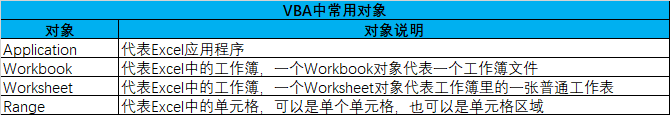1、ScreenUpdating属性

是否控制屏幕更新，False表示关闭屏幕更新，True表示打开屏幕更新

```Sub InputTest()
Cells.ClearContents '清除表中所有数据
Range("A1:A10") = 100
MsgBox "刚才在A1:A10输入数值100，你能看到结果吗？"
Range("B1:B10") = 200
MsgBox "刚才在B1:B10输入数值200，你能看到结果吗？"
End Sub```

```Sub InputTest()
Cells.ClearContents '清除表中所有数据
Application.ScreenUpdating = False '关闭屏幕更新
Range("A1:A10") = 100
MsgBox "刚才在A1:A10输入数值100，你能看到结果吗？"
Range("B1:B10") = 200
MsgBox "刚才在B1:B10输入数值200，你能看到结果吗？"
Application.ScreenUpdating = True '恢复屏幕更新
End Sub```

2、DisplayAlterts属性

是否显示警告对话框，False为不显示，True为显示

```Sub delSht()
Dim sht As Worksheet
For Each sht In Worksheets
If sht.Name = ActiveSheet.Name Then '判断sht是不是活动工作表
sht.Delete '删除sht代表的工作表
End If
Next
End Sub```

3、EnableEvents属性

• 示例1：编写一个程序，当选中工作表的单元格时，自动在单元格中写入该单元格的地址
```Private Sub Worksheet_SelectionChange(ByVal Target As Range)
End Sub```
• 示例2：选中活动单元格，记录对应单元格地址，并将活动单元格向下移动一个单元格
```Private Sub Worksheet_SelectionChange(ByVal Target As Range)
Application.EnableEvents = False '禁用事件
Target.Offset(1, 0).Select '选中活动单元格下面的一个单元格
Application.EnableEvents = True '启用事件
End Sub```

4、WorksheetFunction属性

• 示例1：统计A1:A50单元格中数值大于1000的单元格有多少个？
```Sub CountTest()
Dim mycount As Integer, rng As Range
For Each rng In Range("A1:B50")
If rng.Value > 1000 Then mycount = mycount + 1
Next
MsgBox "A1:B50中大于1000的单元格个数为:" & mycount
End Sub```
• 示例2： 统计A1:A50单元格中数值大于1000的单元格有多少个？使用COUNTIF函数
```Sub CountTest()
Dim mycount As Integer
mycount = Application.WorksheetFunction.CountIf(Range("A1:B50"), ">1000")
MsgBox "A1:B50中大于1000的单元格个数为:" & mycount
End Sub```

5、给Excel梳妆打扮

• Excel工作表界面相关命令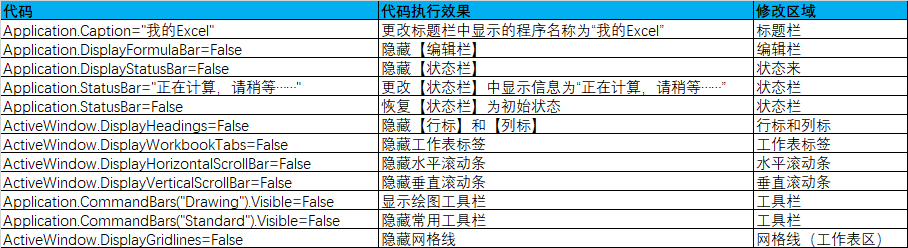• Excel界面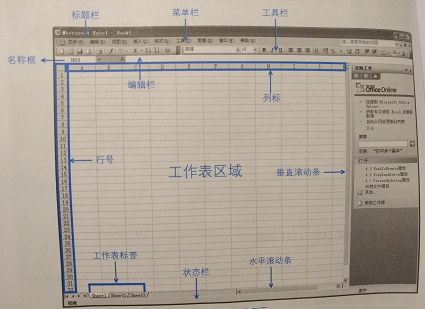6、Application的常用属性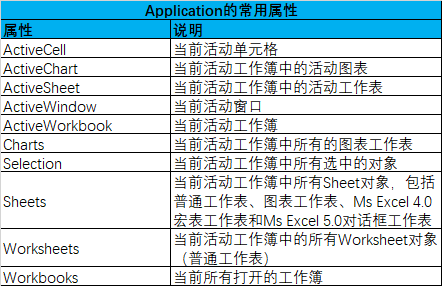Workbook工作簿

Workbooks工作簿集合

1、怎么引用工作簿

2、Workbook名片信息

```Sub wbMsg()
Range("B2") = ThisWorkbook.Name     '返回当前工作簿名称 练习 -副本.xlsm
Range("B4") = ThisWorkbook.FullName '返回当期工作簿带名称的路径 C:\Users\ThinkPad\Desktop\练习 - 副本.xlsm
End Sub```

3、创建工作簿

如果不带任何参数，将创建包含一定数目空白工作表的新工作簿（数目由SheetsInNewWorkbook属性决定）

• 也可以通过参数指定新建工作簿中包含的工作类型

• Excel一共有4种类型的工作表

可以在插入对话框里看到(选中工作表名称——鼠标右键单击——插入——即可显示)，如图（包含参数说明）：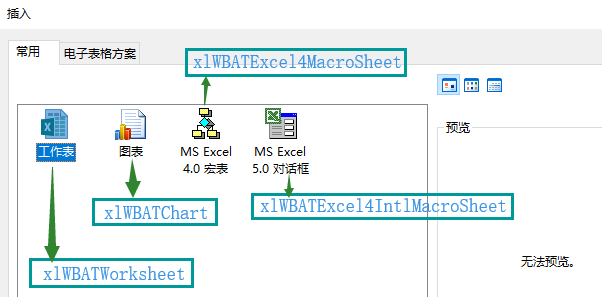4、打开工作簿

使用Workbooks的Open方法（参数名要写含路径的名称）

```Sub OpenFile()
Workbooks.Open Filename:="F:\Book1.xls"
End Sub```

```Sub OpenFile()
Workbooks.Open "F:\Book1.xls"
End Sub```

5、激活工作簿

同事打开多个工作簿，但是同一时间只能有一个窗口是活动的，调用Workbooks对象的Active方法可以激活一个工作簿。

```Sub JhWb()
Workbooks("Book1.xls").Activate '激活工作簿
End Sub```

6、保存工作簿

保存工作簿调用Workbooks的Save方法

```Sub SaveWb()
ThisWorkbook.Save   '保存代码所在的工作簿
End Sub```

如果想将文件另存为一个新的文件，或者第一次保存一个新建的工作簿，就用SaveAs方法。

```Sub SaveWb()
ThisWorkbook.SaveAs Filename:="D:\test.xls"
End Sub```

```Sub SaveWb()
ThisWorkbook.SaveCopyAs Filename:="D:\test.xls"
End Sub```

7、关闭工作簿

关闭工作簿使用Workbooks的Close方法，如果不带参数，则关闭所有打开的工作簿

```Sub CloseWb()
Workbooks.Close '关闭所有打开的工作簿
End Sub```

```Sub CloseWb()
Workbooks("Book1.xls").Close  '关闭Book1.xls
End Sub```

```Sub CloseWb()
Workbooks("Book1.xls").Close savechanges:=True '关闭并保存Book1.xls
End Sub```

```Sub CloseWb()
Workbooks("Book1.xls").Close True '关闭Book1.xls
End Sub```

8、ThisWorkbook与ActiveWorkbook

同是Application对象的属性，同是返回Workbook对象，但二者并不是等同的。

ThisWorkbook是对程序所在的工作簿的引用

ActiveWorkbook是对活动工作簿的引用

```Sub wb()
MsgBox "代码所在的工作簿为：" & ThisWorkbook.Name & Chr(13) _
& "当前活动工作簿为：" & ActiveWorkbook.Name
ActiveWorkbook.Close savechanges:=False
End Sub ```

Worksheet表示一张普通的工作表，Worksheets表示多个Worksheet对象的集合。

1、引用工作表

```Worksheets.Item (1)     '引用工作表里的第一张工作表
Worksheets (1)          '引用工作表里的第一张工作表
Worksheets ("Sheet1")   '引用工作簿里标签名称为"Sheet1"的工作表```

因为代码名称只能在【属性窗口】里修改，不会随着工作表标签名称或索引号的变化而变化。因此，当工作表的索引号或标签名称经常变化时，使用代码名称引用工作表会更方便。

使用代码名称引用工作表，只需直接写代码名称

例如：第一张工作表的A1单元格输入100，代码为：Sheet1.Range("A1")=100

查看工作表的代码名称，可以读取它的CodeName属性，如果想知道活动工作表的代码名称，代码为：

```Sub ShowShtCode()
MsgBox ActiveSheet.CodeName
End Sub```

2、新建工作表

• 不带任何参数，将在活动工作表新建一张工作表

• 可以用参数给新建的工作表指定位置

• 还可以同时插入多张工作表

• 可以同时使用多个参数，不同参数之间用英文逗号隔开
```Sub shtAdd()
End Sub```

在最后一张工作表后插入两张工作表

```Sub shtAdd()
'在最后一个工作表后插入两张工作表
End Sub ```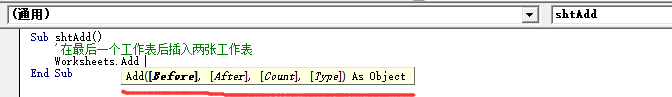3、更改工作表标签名称

• 更改工作表标签名称，设置工作表Name属性

Worksheets(2).Name="工资表"   '更改第二张工作表的标签名称为“工资表”

•  新建工作表时在程序中更改标签名称
```Sub shtAdd()
ActiveSheet.Name = "工资表"
End Sub```
• 新建工作表同时指定它的标签名称
```Sub shtAdd()
'在第一张工作表前插入一个名称为“工资表”的工作表
End Sub```
• 如果同时添加多张工作表（即Count参数值大于1），并不能使用一句代码同时命名

4、删除工作表

Worksheets("Sheet1").Delete   '删除Sheet1工作表

5、激活工作表

激活工作表可以使用Activate方法和Select方法

Worksheets(1).Activate '激活第一张工作表
Worksheets(1).Select '激活第一张工作表

6、复制工作表

复制工作表使用Copy方法

```Sub shtCopy()
'这里的工作表名称一定要存在，否则执行会报错
Worksheets("工资条").Copy  '不带参数   复制工作表，同时新建工作簿用于存放copy来的工作表（未保存状态）
Worksheets("工资条").Copy before:=Worksheets("Sheet1") '带参数  复制工作表，存放在当前工作簿的工作表Sheet1之前
Worksheets("工资条").Copy after:=Worksheets("Sheet1") '带参数   复制工作表，存放在当前工作簿的工作表Sheet1之后
End Sub```

7、移动工作表

移动工作表与复制工作表类似，使用方法Move

```Sub shtMove()
Worksheets("工资条").Move   '不指定参数，将把工作表移动到新的工作簿中（新建工作簿）
Worksheets("工资条").Move before:=Worksheets("Sheet1") '复制工作表，存放在当前工作簿的工作表Sheet1之前
Worksheets("工资条").Move after:=Worksheets("Sheet1") '复制工作表，存放在当前工作簿的工作表Sheet1之后
End Sub```

8、隐藏和显示工作表

```'以下这三行代码作用一样，等同于从【格式】菜单中隐藏工作表
Worksheets("工资条").Visible = False
Worksheets("工资条").Visible = xlSheetHidden
Worksheets("工资条").Visible = 0```

```Worksheets("工资条").Visible = xlSheetVeryHidden
Worksheets("工资条").Visible = 2```

```Worksheets("工资条").Visible = True
Worksheets("工资条").Visible = xlSheetVisible
Worksheets("工资条").Visible = 1
Worksheets("工资条").Visible = -1```

9、获取工作表的数目

```Dim mycount%
mycount=Worksheets.Count ```

10、Sheets与Worksheets

•  不同的命令，返回相同的结果
```Sheets(2).Name
Worksheets(2).Name

Sheets.Count
Worksheets.Count```
• 分别代表两种不同的集合

Excel里共有4中不同类型的工作表，Sheets表示公祖不里所有类型的工作表的集合，而Worksheets只表示普通工作表的集合。

1、Worksheet(或Range)对象的Range属性

• 引用单元格并赋值

Worksheets("sheet1").Range("A1").Value=50

```Sub rng()
Range("A1:A10").Value = 200 '在活动工作表的A1:A10输入值为200

Dim n As String
n = "B1:B10"
Range(n) = 100 '在活动工作表的B1:B10输入值为100
End Sub ```
• 通过设置“单元格区域名称”调用Range
```Sub rng()
Range("date").Value = 200
End Sub```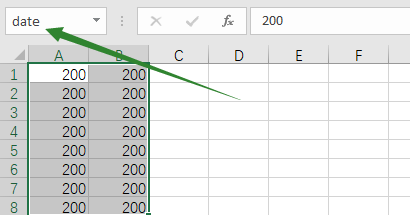• 引用多个不连续的区域，用逗号隔开
```Sub rng()
Range("A1:A10,A4:E6,C3:D9").Value = 200
End Sub```
• 用空格而不是逗号，则表示选中区域交集部分
```Sub rng()
Range("A1:B10 A4:D9").Value = 200
End Sub```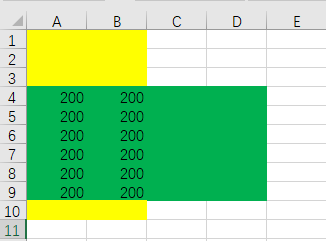2、Worksheet(或Range)对象的Cells属性

•  指定单元格
```Sub shtCells()
ActiveSheet.Cells(3, 4).Value = 20  '在第3行，第4列香蕉的单元格输入20
ActiveSheet.Cells(3, "D").Value = 30 '在第3行，第D列相交的单元格输入30

Range("B3:F9").Cells(2, 3) = 40 '在区域“B3:F9”区域中的第2行，第3列相交的单元格，即D4
ActiveSheet.Cells(2).Value = 50 '在活动工作表的第二个单元格输入50,这里使用的数字2是单元格序号，序号是按照单元格区域内由左向右递增

'选中活动工作表的A1:E10
Range(Cells(1, 1), Cells(10, 5)).Select

'以下两个语句等价
Range("A1", "E10").Select
Range(Range("A1"), Range("E10")).Select
End Sub```
• 全部单元格
```Sub shtCells()
ActiveSheet.Cells.Select  '选中活动工作表的所有单元格
Range("B3:E9").Select '选中活动工作表中B3:E9单元格区域
End Sub```
• 更简短的快捷方式
```Sub shtCells()
[A1] = 10
[A1:B10] = 20
[B3:D10 A4:G8] = 100 '公共交叉区域，如果两个区域参数没有逗号，表示一个参数，而参数表示的区域没有交集的话会报错
[A1:A10,C1:C10,E1:E10] = 200 '合并区域
[area] = 300 '名称are代表单元格，即单元格名称为area
End Sub```

[]是Application对象的Evaluate方法的简写形式，这种简写形式非常适合饮用一个固定的Range对象，但是因为不能再方括号中使用变量，所以这种引用方式缺少灵活性。

4、其他获取单元格的方式（除了Range、Cells外）—Rows

```ActiveSheet.Rows '选中活动工作表的所有行
ActiveSheet.Rows(3).Select '选中活动工作表的第3行
ActiveSheet.Rows("3:3").Select '选中活动工作表的第3行
ActiveSheet.Rows("3:5").Select '选中活动工作表的第3行到第5行
Rows("3:10").Rows("1:1").Select '选中第3行到第10行区域内的第一行```

5、其他获取单元格的方式（除了Range、Cells外）—Columns

```ActiveSheet.Columns  '选中活动工作表的所有列
ActiveSheet.Columns (6) '选中活动工作表中的第6列
ActiveSheet.Columns ("F:G") '选中活动工作表中的F至G列
Columns("B:G").Columns("B:B").Select '选中B:G区域中的第2列```

6、Application的Union方法

Union方法像一支强烈的粘合剂，将不连续的多个单元格区域粘在一起，可以同时对其进行操作。

```Sub rngUnion()
Application.Union(Range("A1:A10"), Range("D1:D5")).Select '入参至少为2个区域，至多30个区域，区域之间用逗号分隔
Union(Range("A1:A10"), Range("D1:D5")).Select  'application可以省略不写
End Sub```

7、Range对象的Offset属性

Offset属性用来基于基于单元格的位置移动

Offset(x,y)两个参数，x表示行移动，即x>0表示向下移动，x<0表示向上移动；y表示列移动，即y>0表示向右移动，y<0表示向左移动。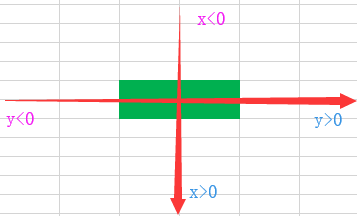```Sub rngOffset()
Range("A1").Offset(2, 3).Value = 500 '基于“A1”单元格，向下移动2行，向右移动3列
Range("C5:D6").Offset(-3, 0).Select '在“C5：D6”区域的基础上，向上移动3行，列方向参数为0，不移动。
End Sub```

8、Range对象的Resize属性

使用Range对象的Resize属性扩大或缩小指定的单元格区域，得到一个新的单元格区域。

Resize共有两个参数，第一个参数确定新区域的行数，第二个参数确定新区域的列数，两个参数的值都是正整数，最小为1.

```Sub rngResize()
'将B2单元格扩大为B2:E6
Range("B2").Resize(5, 4).Select

'将B2：E6单元格缩小为B2：B3，新区域以B2单元格为最左上角单元格
Range("B2:E6").Resize(2, 1).Select
'上句等同于
Range("B2:E6").Cells(1).Resize(2, 1).Select
End Sub```

9、Worksheet对象的UsedRange属性

UsedRange属性返回工作表中已经使用的单元格围成的矩形区域（不管这些区域间是否有空行，空列或空单元格）。

```Sub rngUsed()
ActiveSheet.UsedRange.Select
End Sub```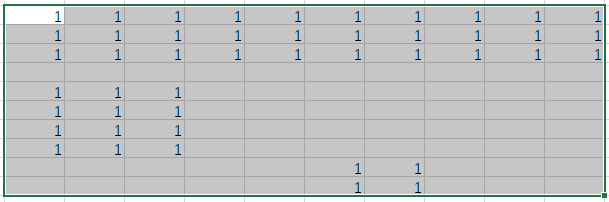10、Range对象的CurrentRegion属性

CurrentRegion返回当前区域，即以空行和空行的组合为边界的区域

```Sub rngUsed()
Range("D3").CurrentRegion.Select
End Sub```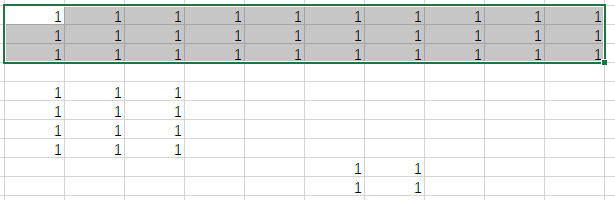11、Range对象的End属性

End属性返回当前区域结尾处的单元格，等同于在源单元格按<End+方向键（上下左右）>得到的单元格。

```Sub rngEnd()
Range("E5").End(xlUp).Select
End Sub```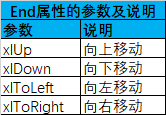```Sub rngEnd()
'取第一个单元格，如果非空则向下移动一个单元格，否则不移动。对新单元格进行赋值
Dim c As Range
Set c = ActiveSheet.Range("A65536").End(xlUp)
If c.Value <> "" Then
Set c = c.Offset(1, 0)
End If
c.Value = "张青"
End Sub

Sub rngUsed()
'取使用区域内行数增加1，对该行的A列进行赋值
Dim xrow As Long
xrow = ActiveSheet.UsedRange.Rows.Count + 1
Cells(xrow, "A").Value = "张青"
End Sub

Sub rngCurr()
'取当前区域内行数增加1，对该行的A列进行赋值
Dim xrow As Long
xrow = Range("A1").CurrentRegion.Rows.Count + 1
Cells(xrow, "A").Value = "张青"
End Sub```

1、单元格内容-Value

```Range("A1:B2").Value = "abc"
Range("A1:B2") = "abc"  'Value是Range的默认属性，在给区域赋值时可以省略。```

2、单元格个数-Count

```Range("B4:F10").Count   '统计单元格数量
ActiveSheet.UsedRange.Rows.Count '统计活动单元格的行数
ActiveSheet.UsedRange.Columns.Count  '统计活动单元格的列数```

4、选中单元格-Active与Select

```ActiveSheet.Range("A1:B10").Select
ActiveSheet.Range("A1:B10").Activate```

5、选择性清除单元格-Clear

```Range("B2:B15").Clear '清除B2:B15单元格所有内容（包括批注、内容、注释、格式等）
Range("B2:B15").ClearContents '清除B2:B15单元格内容
Range("B2:B15").ClearFormats '清除B2:B15单元格格式```

6、复制&粘贴单元格区域-Copy&Paste

• 录制复制和粘贴的宏内容如下：
```Sub Macro1()
Range("A1").Select
Selection.Copy
Range("C1").Select
ActiveSheet.Paste
End Sub```
• 但在执行复制或者粘贴操作之前并不需要选中单元格，所以代码可以简化为：
```Sub Macro1()
Range("A1").Copy Range("C1") 'A1是源单元格，C1是目标单元格
End Sub```
• 带参数的复制-Destination
```Sub Macro1()
Range("A1").Copy Destination:=Range("C1") 'A1是源单元格，C1是目标单元格,Destination是目标
End Sub```
• 带参数的复制-CurrentRegion

要复制的单元格区域不能确定大小，可以只指定一个单元格作为目标区域的最左上角单元格

```Sub Macro1()
Range("A1").CurrentRegion.Copy Range("C1") 'A1是源单元格，C1是目标单元格,Destination是目标
End Sub```
• 想粘贴源区域的数值（以下两个式子等价）
```Sub rngCopyValue_1()
Range("A1:A10").Copy
Range("F1:F10").PasteSpecial Paste:=xlPasteValues '仅粘贴数值
End Sub
Sub rngCopyValue_2()
Range("A1:A10").Value = Range("F1:F10").Value
End Sub```

7、剪切单元格-Cut

```Sub rngCut()
Range("A1:A5").Cut Destination:=Range("G1") '把A1:A5剪切到G1:G5，这里G1表示以G1为左上角第一个单元格的区域
Range("F6:F10").Cut Range("G6") '把F1:F10剪切到G6:K10，参数Destination可以省略
End Sub```

8、删除单元格-Delete

Delete有4个选项，分别对应如下参数：

```Range("B5").Delete Shift:=xlToLeft '删除B5单元格，删除后右侧单元格左移
Range("B5").Delete Shift:=xlUp '删除B5单元格，删除后下方单元格上移
Range("B5").EntireRow.Delete '删除B5单元格所在的行
Range("B5").EntireColumn.Delete '删除B5单元格所在的列```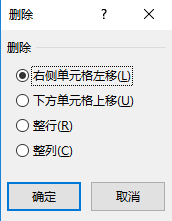9、单元格名称，Names集合

Excel中定义的名称就是给单元格区域（或数值、常量、公式）取的名字，一个自定义的名称及时一个Name对象，Names是工作簿中定义的所有名称的集合。

• 新建名称

```'Add新建名称的方法，RefersToR1C1表示使用R1C1引用样式

R5C[-2]说明：R后面的数值表示行号，C后面的数值表示列号，[]中括号表示相对引用，默认是绝对引用，相对应用时R>0表示向下移动，C>0表示向右移动

RC：对活动单元格下方的第二行与右边的第3列相交的单元格的引用

R2C3：对工作表中第二行与第3列相交的单元格的引用

• 另一种单元格引用方式：A1样式引用
```'Add新建名称的方法，RefersToR1C1表示使用A1引用样式,\$表示相对绝对引用，将把活动单元格当做A1单元格
• 定义名称更简单的方式
`Range("A1:C10") = "date"`
• 怎样引用名称
```ActiveWorkbook.Names("date").Name = "姓名"
ActiveWorkbook.Names("姓名").Name = "张三"```
• 也可以使用名称索引引用名称
```Sub UseName()
Dim i, mx As Integer
mx = ActiveWorkbook.Names.Count '统计一共有多少个单元格
For i = 1 To mx
activateworkbook.Names(i).Visible = False '隐藏名称
Next
End Sub```

10、单元格批注，Comment对象

• 给单元格增加批注
`Range("B5").AddComment Text:="我用VBA新建的批注"`
• 怎么知道单元格是否有批注
```Sub wbComment()
If Range("B5").Comment Is Nothing Then '判断是否存在Comment对象
MsgBox "B5单元格中没有批注"
Else
MsgBox "B5单元格中已有批注"
End If
End Sub```
• 操作批注
```Sub operComment()
Range("B5").Comment.Visible = False '隐藏B5单元格批注
Range("B5").Comment.Delete '删除B5单元格批注
End Sub```

11、给单元格化妆

•  设置字体-Font
```Sub FontSet()
With Range("A1:L1").Font
.Name = "宋体" '设置字体为宋体
.Size = 12 '设置字号为12号
.Color = RGB(255, 0, 0) '设置字体颜色为红色
.Bold = True '设置字体加粗
.Italic = True '设置字体倾斜显示
.Underline = xlUnderlineStyleDouble 'feud文字添加双下划线
End With
End Sub```
• 给单元格增加底纹-Interior
```Sub InteriorSet()
Range("A1:L1").Interior.Color = RGB(255, 255, 0) '增加黄色底纹
End Sub```
• 给表格设置表框
```Sub InteriorSet()
With Range("A1").CurrentRegion.Borders
.LineStyle = xlContinuous '设置单线边框
.Color = RGB(0, 0, 255) '设置边框颜色
.Weight = xlHairline '设置边框线条样式
End With
End Sub```
• 其他设置

可以在“单元格格式”对话框中进行其他设置，如果想用代码实现而不知道代码怎么写，可以手动操作，用宏录制器录下它。

1、编写一个程序，按要求创求的一个新的工作簿，并把它保存到指定的文件夹。

```Sub wbAdd()
'程序创建“员工花名册”工作簿，保存在本工作簿所在的文件夹中
Dim wb As Workbook, sht As Worksheet '定义一个Workbook对象和一个Worksheet对象
Set sht = wb.Worksheets(1)
With sht
.Name = "花名册"  '修改第一张工作表的标签名称
.Range("A1:F1") = Array("序号", "姓名", "性别", "出生年月", "参加工作时间", "备注") '设置表头
End With
wb.SaveAs ThisWorkbook.Path & "\员工花名册.xls" '保存新建的工作表到本工作簿所在的文件夹中
ActiveWorkbook.Close '关闭新建的工作簿
End Sub```

2、判断工作簿是否打开

• 工作簿是否打开判断
```'判断"成绩表.xls"工作簿是否打开
Sub isWbOpen()
Dim i As Integer
For i = 1 To Workbooks.Count
If Workbooks(i).Name = "成绩表.xls" Then
MsgBox "文件已打开"
Exit Sub '如果找到该文件，退出过程
End If
Next
MsgBox "文件没有打开"
End Sub```
•  工作表是否打开判断
```'判断打开的工作表中是否含“一年级”，有则移动到第一个位置，否则在第一个位置创建
Sub isShtOpen()
Dim sht As Worksheet
For Each sht In Worksheets
If sht.Name = "一年级" Then
sht.Move before:=Worksheets(1)
'MsgBox "已经打开"
Exit Sub
End If
Next
End Sub```

另一种写法：

`'判断打开的工作表中是否含“一年级”，有则移动到第一个位置，否则在第一个位置创建`
```Sub isShtOpen()
On Error Resume Next
If Worksheets("一年级") Is Nothing Then
Else
Worksheet("一年级").Move before:=Worksheets(1)
'MsgBox "已经打开"
End If
End Sub```

3、判断工作簿是否存在

```Sub isExistWb()
'判断本工作簿所在的文件夹中是否存在“员工花名册.xls”
Dim fil As String
fil = ThisWorkbook.Path & "\员工花名册.xls"
If Len(Dir(fil)) > 0 Then
MsgBox "工作簿已经存在"
Else
MsgBox "工作簿不存在"
End If
End Sub```

4、向未打开的工作簿中录入数据

```Sub WbInput()
'在本工作簿所在的文件夹下“员工花名册”里添加一条记录
Dim wb As String, xrow As Integer, arr
wb = ThisWorkbook.Path & "\员工花名册.xls"
Workbooks.Open (wb)
With ActiveWorkbook.Worksheets(1)
xrow = .Range("A1").CurrentRegion.Rows.Count + 1
arr = Array(xrow - 1, "张娇", "女", "#7/8/1987#", "#9/1/2010#", "10年新招")
.Cells(xrow, 1).Resize(1, 6) = arr
End With
ActiveWorkbook.Close savechanges:=True
End Sub```

5、隐藏活动工作表外的所有工作表

```Sub ShtVisible()
'隐藏活动工作表外的所有工作表
Dim sht As Worksheet
For Each sht In Worksheet
If sht.Name <> ActiveSheet.Name Then
sht.Visible = xlSheetVeryHidden '深度隐藏，不能通过“格式”菜单显示它
End If
Next
End Sub```

6、批量新建工作表

```Sub shtAdd()
'一张成绩表中保存不同班级的数据，需要以班级名命名
'根据C列的班级名新建不同的工作表
Dim i As Integer, sht As Worksheet
i = 2
Set sht = Worksheets("成绩表")
Do While sht.Cells(i, "C") <> ""
ActiveSheet.Name = sht.Cells(i, "C").Value
i = i + 1
Loop
End Sub```

7、批量对数据分类

```Sub fenLei()
'把成绩按班级分到各个工作表中
Dim i As Long, bj As String, rng As Range
i = 2
bj = Cells(i, "C").Value
Do While bj <> ""
'将分表中A列第一个空单元格赋给rng
Set rng = Worksheets(bj).Range("A65536").End(xlUp).Offset(1, 0)
Cells(i, "A").Resize(1, 7).Copy rng '将记录赋值到对应的工作表中
i = i + 1
bj = Cells(i, "C").Value
Loop
End Sub```

```Sub shtClear()
Dim sht As Worksheet
For Each sht In Worksheets
If sht.Name <> "成绩表" Then
sht.Range("A2:G65536").ClearContents
End If
Next
End Sub```

8、将工作表保存为新工作簿

```Sub SaveToFile()
'把各个工作表以单独的工作簿文件保存在本工作簿所在的文件夹下的“班级成绩表”文件夹下
Application.ScreenUpdating = False '关闭屏幕更新
Dim folder As String
folder = ThisWorkbook.Path & "\班级成绩表"
'如果文件夹不存在，则新建文件夹
If Len(Dir(folder, vbDirectory)) = 0 Then mkdir folder
Dim sht As Worksheet
For Each sht In Worksheets
sht.Copy
ActiveWorkbook.SaveAs folder & "\" & sht.Name & ".xlsx"
ActiveWorkbook.Close
Next
Application.ScreenUpdating = True
End Sub```

```Sub 自动拆分工作表()
'
' 自动拆分工作表 宏
'
' 快捷键: Ctrl+m
'
'把各个工作表以单独的工作簿文件保存在本工作簿所在的文件夹下的“拆分工作簿”文件夹下
Application.ScreenUpdating = False '关闭屏幕更新
Dim folder As String
folder = Application.ActiveWorkbook.Path & "\拆分工作簿"
'folder = ThisWorkbook.Path & "\拆分工作簿"
'如果文件夹不存在，则新建文件夹
If Len(Dir(folder, vbDirectory)) = 0 Then MkDir folder
Dim sht As Worksheet
For Each sht In Worksheets
sht.Copy
ActiveWorkbook.SaveAs folder & "\" & sht.Name & ".xlsx"
ActiveWorkbook.Close
Next
Application.ScreenUpdating = True

End Sub```

9、快速合并多表数据

```Sub HeBing()
'把各班级成绩表合并到“总成绩”工作表中
Rows("2:25536").Clear '删除原有记录
Dim sht As Worksheet, xrow As Integer, rng As Range
For Each sht In Worksheets  '遍历工作簿中所有工作表
If sht.Name <> ActiveSheet.Name Then
Set rng = Range("A65536").End(xlUp).Offset(1, 0) '获得A列第一个空单元格
xrow = sht.Range("A1").CurrentRegion.Rows.Count - 1 '记录分表中记录条数
sht.Range("A2").Resize(xrow, 7).Copy rng '粘贴记录到汇总表
End If
Next
End Sub```

10、汇总同文件夹下多个工作簿数

```Sub HzwWb()
'把目前下各个工作簿的信息汇总到同文件夹下的另一个工作簿的同一张工作表里
Dim r, c As Long
r = 1 '表头的行数
c = 8 '表头的列数
Range(Cells(r + 1, "A"), Cells(65536, c)).ClearContents '清空汇总表中原数据
Application.ScreenUpdating = False '关闭屏幕更新
Dim FileName As String, wb As Workbook, sht As Worksheet, Erow As Long, fn As String, arr As Variant
FileName = Dir(ThisWorkbook.Path & "\" & "*.xlsx")
Do While FileName <> ""
If FileName <> ThisWorkbook.Name Then '判断文件是否是本工作簿
Erow = Range("A1").CurrentRegion.Rows.Count + 1 '取得汇总表中第一条空行行号
fn = ThisWorkbook.Path & "\" & FileName
Set wb = GetObject(fn) '将fn代表的工作簿对象赋给变量
Set sht = wb.Worksheets(1) '汇总的是第一张工作表
'将数据表中的记录保存在arr数组里
arr = sht.Range(sht.Cells(r + 1, "A"), sht.Cells(65536, "B").End(xlUp).Offset(0, 8))
'将数组arr中的数据写入工作表
Cells(Erow, "A").Resize(UBound(arr, 1), UBound(arr, 2)) = arr
wb.Close False
End If
FileName = Dir '用Dir函数取得其他文件名，并赋值给变量
Loop
Application.ScreenUpdating = True '恢复屏幕更新
End Sub```

11、为工作表建立目录

```Sub mkdir()
'为工作簿中所有工作表建立目录
Rows("2:65536").ClearContents
Dim sht As Worksheet, irow As Integer
irow = 2
For Each sht In Worksheets '遍历工作表
Cells(irow, "A").Value = irow - 1 '写入序号
'写入工作表名，并建立超链接
SubAddress:="'" & sht.Name & "'!A1", TextToDisplay:=sht.Name
irow = irows + 1 '行号加1
Next
End Sub```

https://www.cnblogs.com/wzh313/articles/9739085.html